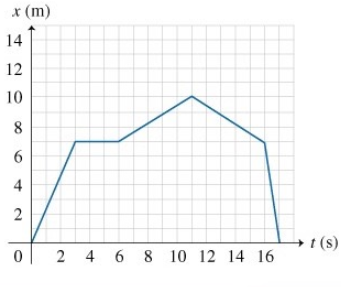# Problem: Find the object's speeds v1, v2, and v3 at times t1 = 2.0 s, t2 = 4.0 s, and t3 = 13 s. Express your answers in meters per second to two significant figures, separated by commas.

###### FREE Expert Solution

Velocity:

$\overline{){\mathbf{v}}{\mathbf{=}}\frac{\mathbf{∆}\mathbf{x}}{\mathbf{∆}\mathbf{t}}}$

v1

At t= 2.0 s

82% (168 ratings)###### Problem Details

Find the object's speeds v1, v2, and v3 at times t1 = 2.0 s, t2 = 4.0 s, and t3 = 13 s. Express your answers in meters per second to two significant figures, separated by commas.